The effectiveness of the use of the android-based carom games comic integrated to discovery learning in improving critical thinking and mathematical representation abilities

THE EFFECTIVENESS OF THE USE OF THE ANDROID-BASED CAROM GAMES COMIC INTEGRATED TO DISCOVERY LEARNING IN IMPROVING CRITICAL THINKING AND MATHEMATICAL REPRESENTATION ABILITIES

Universitas Negeri Yogyakarta (Indonesia)

Accepted March 2021

Abstract

This research aims to reveal the effectiveness of the use of the Android-based carom games comic integrated to discovery learning (MIKIMOM) in improving critical thinking and mathematical representation in the teaching of physics. It is quantitative research with the quasi experimental research design. It was conducted in two phases, with the first phase for empirical try out of the test and second phase for the application of MIKIMOM to reveal the effectiveness of the developed media in teaching. The empirical try out was conducted to 261 grade XI MIA students. The application of the media was conducted to 32 students of grade X MIA 3 State Senior High School (SSHS) 2 Banguntapan as an experimental class using MIKIMOM and 32 students of grade X MIA 4 State Senior High School (SSHS) 2 Banguntapan as an experimental class using the Powerpoint (PPT) media. The data were collected using a critical thinking and mathematical representation test which was valid and reliable according to the empirical testing. The data were analyzed using the QUEST program to see the validity and reliability of the test items, and the MANOVA test to see the effectiveness of the media. The research finding shows that MIKIMOM is effective in improving the critical thinking and mathematical representation of the students with the scores of 0.287 (large effect size) and 0.179 (medium effect size).

Keywords – Critical thinking, mathematical representation, MIKIMOM.

 Rahayu, M.S.I., & Kuswanto, H. (2021). The effectiveness of the use of the android-based carom games comic integrated to discovery learning in improving critical thinking and mathematical representation abilities. Journal of Technology and Science Education, 11(2), 270-283. https://doi.org/10.3926/jotse.1151

----------

1. 1. Introduction

Critical thinking is considered as an important component in the activities of a student as a scientist (Viennot & Nicolas, 2018; Wahyudi, Verawati, Ayub & Prayogi, 2019). This is due to the fact that critical thinking will encourage students to reason, discuss, express their reason, and draw conclusions. Critical thinking enables one to distinguish facts from opinions and to evaluate and assess the truth of available evidence (El Soufi & See, 2019). However, the critical thinking ability of students is low (Puspita, Kaniawati & Suwarma, 2017; Sutrisno, Handayanto, Supriyana & Laksmisari, 2018). They still find it difficult to comprehend all materials and they can comprehend very little about certain concepts in every topic of physics teaching (Karim, Saepuzaman & Sriyansyah, 2015; Budiarti, Rusnayati, Siahaan & Wijaya, 2018) and therefore, they have lacks of patterns. The difficulties in understanding the materials include the difficulties in understanding basic concepts, distinguishing symbols, and relating one concept with other concepts (Yuliati, Fauziah & Hidayat, 2018). In addition, the students cannot understand concepts and do analyses to solve problems because they are often faced with problems which require mathematic equation so that they also need to have mathematical representation ability (Siswanto, Susantini & Jatmiko, 2012; Bego, Chastain, Pyles & DeCaro, 2018).

Mathematical representation is a way to show a condition using a mathematical model (Park & Choi, 2013; Purwadi, Sudiarta & Suparta, 2019). It is used to observe prediction and assumption of a problem using reasoning skill so that the students can understand, make, and evaluate assumptions and arguments mathematically and systematically (Cartrette & Bodner, 2010; Nor’ain Mohd & Chinnappan, 2016; Doran, 2017). In general, mathematical representation takes the form of equation and formula (Namdar & Shen, 2017) and the combination of mathematical symbols and structure which use numbers, variables, and equation (Jaccard & Jacoby, 2010; Priyadi, Kuswanto & Sumarna, 2020). This equation in physics is called physics language (Docktor & Mestre, 2014). The equation can be used for determining the solution to a problem through the manipulation of the symbol of numbers of an identified variable so that it can be used as a proof of a solution process (Kuo, Hull, Gupta & Elby, 2012; Hau, Marwoto & Putra, 2018). In other words, mathematical representation is a mathematical equation which reveals a concept (Redish & Kuo, 2015). It can change an abstract concept to become concrete by using equations or graphs, so that teaching becomes more meaningful (Supandi, Waluya, Rochmad, Suyitno & Dewi, 2018). However, the students’ representation ability is still low. They find difficulties in mathematic basic operational concepts, placing positive and negative symbols in numbers that results in wrong calculation (Jitendra, Nelson, Pulles, Kiss & Houseworth, 2016), using arithmetic symbols in problem solution (Sari, Dahrim & Rosjanuardi, 2018), and determining variables for making equations (Redish & Kuo, 2015).

Based on the above analysis, there needs for learning that can help students improve their critical thinking and mathematical representation. Mathematical representation and critical thinking abilities can be improved in many ways, one of which is by applying a correct model and media. The teaching model which can be applied is discovery learning (Minarni, Napitupulu & Husein, 2016).

Discovery learning is a teaching model for active students because they are obliged to discover their own concept with the help of the provided stimuli. The experience in discovering and investigating by themselves will make their memory of a concept long lasting and not easily forgotten (Hosnan, 2014; Martaida, Bukit & Ginting, 2017). The use of discovery learning facilitates the students’ critical thinking in teaching because this teaching model has the steps which can encourage them to interact and make ideas based on observation, experiment, and enquire (Sulistiani, Waluya & Masrukan, 2018). The discovery learning steps in teaching include making stimuli, identifying problems, collecting data, verifying data, and drawing conclusion (In’am & Hajar, 2017). The use of discovery learning can also bring up curiosity to solve problems well and to think critically (Ramdhani, Usodo & Subanti, 2017). Curiosity affects the motivation to learn so that students will be motivated to find the solution to an existing problem by thinking critically (Isnaningsih & Bimo, 2013). This kind of teaching when collaborated with teaching media can result in interesting condition, make students more active, and improve their motivation to learn so that their learning achievement will improve (Permatasari, Soegiyanto & Usodo, 2019)

One of the media which can be used to support teaching representation which is interesting and can facilitate students to learn is Android-based physics comics (Irwandani & Juariah, 2016; Rahayu & Kuswanto, 2020). Comic is a combination of pictures and texts which can increase the effectiveness of education because it is a teaching aid which can convey information effectively and efficiently (Guérin, Rigaud, Bertet & Revel, 2017; Mutia, Gani & Syukri, 2020). Learning through comics enables students to express new ideas so that it is not boring (Cahyono, Isnandar & Usodo, 2019). Android-based media can also be used for learning physics anytime and anywhere (Raras & Kuswanto, 2019; Maghfiroh, Kuswanto & Susetyo, 2020). They can replace packaged books because they already contain illustrating video, materials, examples of test items, discussion, and practice test (Nikmah, Haroky, Jumadi, Wilujeng & Kuswanto, 2019). They can be integrated into a teaching model or approach (Rahayu & Kuswanto, 2020). Besides, they will also facilitate learning processes, especially in actualizing abstract concepts through concrete everyday lives (Yulianti et al., 2016; Rina, 2020) such as carom games.

The car om game is one of the traditional games which use a square table having one hole in each diagonal corner (Atmaja, Jonemaro & Arwani, 2017). This game applies many strategies and analyses of the condition to put carom pieces (pucks) in pockets accurately and win the game (Asisningtyas & Suryanti, 2017). In fact, some conditions and events in carom games are related to physics teaching materials, i.e. the concept of momentum and impulse. Therefore, this research applies an Android-based carom games comic integrated to discovery learning (MIKIMOM = comic impulse momentum) and reveals it is the effectiveness in improving critical thinking and mathematical representation abilities in the teaching of physics.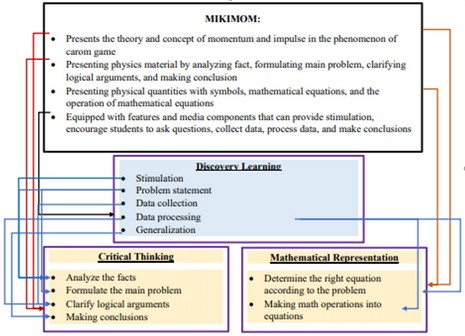Figure 1. Conceptualization of MIKIMOM as a learning media that integrated with discovery
learning to improve critical thinking and mathematical representation abilities

2. Research Method

2.1. Type of Research

This research is a quantitative study with the quasi experimental research design. It uses two classes: experimental class and control class. The experimental class is the class that is given treatment of the use of an Android-based carom games comic integrated to discovery learning (MIKIMOM), while the control class is the class taught by using the power points (PPT). PPT is used in the control class because PPT is a conventional learning media that is often used by physics teachers at State Senior High School (SSHS) 2 Banguntapan to delivering physics material. The research design can be seen in Table 1.

 Group Pretest Treatment Posttest Y1 Y2 Y1 Y2 Experimental Class T1 T1 X1 T2 T2 Control Class T1 T1 X2 T2 T2

Table 1. Research design

Notes

T1: Pretest

T2: Posttest

Y1: Critical thinking

Y2: Mathematical representation

X1: Application of Android-based carom games comic integrated to discovery learning (MIKIMOM)

X2: Physics teaching by using PPT

2.2. Research Sample

Administering the critical thinking and mathematical representation ability test in the materials about momentum and impulse to 261 grade XI students aiming at revealing the feasibility of the test in terms of validity and reliability. The students consist of those of State Senior High School (SSHS) 1 Plered (two classes), SSHS 2 Banguntapan (two classes), SSHS 1 Ngemplak (two classes), SSHS 1 Ngaglik (three classes), and SHS Bina Umat (two classes). All of the students undertook the momentum and impulse materials when they were in grade X. Experiment MIKIMOM at SSHS 2 Banguntapan aiming at revealing the effectiveness of the comic in improving critical thinking and mathematical representation abilities. The population for testing the effectiveness of MIKIMOM was 180 students of grade X at SSHS 2 Banguntapan. The sample selection was carried out using the cluster random sampling technique so that the experimental class was obtained as many as 32 students MIA 3 and the control class as many as 32 students X MIA 4. Students as the research sample consisted of male and female with the average of students being 15-16 years old, students come from medium socio-economic conditions so that all of students have android as their source and learning media. Testing the effectiveness of comics is by using a pretest and posttest with a distance of giving questions for two weeks (two meetings).

2.3. Research Instrument and Procedure

The instruments used in this research consist of a teaching instrument and data collecting instrument. The teaching instrument is in the form of lesson plans, MIKIMOM, and PPT. The data collecting instrument is an essay test of critical thinking and mathematical representation abilities in momentum-impulse materials, each of which consists of four items as shown in Table 2 below.

 Basic competence Indicators of critical thinking ability Item no Indicators of test items Applying the concept of impulse and momentum, as well as Momentum Immunity Law in everyday life Analyzing facts Formulating problems Clarifying logical arguments Drawing conclusions 1 Presented with a description of a child kicking a wooden table and a sofa of a different material so that he experiences different pains, the students can analyze facts, formulate problems, clarify logical arguments, and draw conclusion based of the concept of impulse in the event. 2 Presented with a description of a teacher throwing a ball at students and then giving alternatives so that the students can catch the ball easily, students can analyze facts, formulate problems, clarify logical arguments, and draw conclusion based of the concept of impulse in the event. 3 Presented with a description and picture of stationary carom pieces (pucks) which are then struck with a certain strength and interval, students can analyze facts, formulate problems, clarify logical arguments, and draw conclusion on the extent of the change occurring to the pucks. 4 Presented with a description of colliding pucks which then move with different directions and speeds after the collision, students can analyze facts, formulate problems, clarify logical arguments, and draw conclusion on the type of collision that has occurred.

Table 2. Indicators of critical thinking in the topic of momentum and impulse

 Basic competence Indicators of mathematical representation Item no Indicators of test items Applying the concept of impulse and momentum, as well as Momentum Immunity Law in everyday life Determining a correct equation according to a problem 1 Presented with a description of two children striking the same puck but with different speeds, students can determine a correct equation for the amount of momentum of each child. Doing mathematical operation into an equation 2 Presented with a description of a colliding striker with stationary target pucks, students can determine the speed of the striker after the collision. Doing mathematical operation into an equation 3 Presented with a description of a pingpong ball falling from a certain height and bouncing on the floor, students can determine the restitution of the bounce. Determining a correct equation based on a problem 4 Presented with a description of a penalty kick, students can determine the equation of the duration of the foot touching the ball.

Table 3. Indicators of mathematical representation in the topic of momentum and impulse

This study uses the quasi experimental research design. The following is the procedure taken, as shown in Figure 2.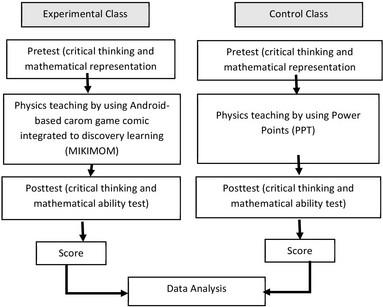Figure 2. Research implementation scheme

2.4. Data Analysis

The analysis of the data on the test item feasibility was conducted using the QUEST program in order to reveal the item fitness (reliability and validity) as the requirement for the items to be used. The item reliability can be known from the summary of item estimates and summary of case estimates in the QUEST program output ranging from 0.6 to 0.8, while the item validity can be known from the Infit Mean Square (INFIT MNSQ) which ranges from 0.77 to 1.30, where an item is considered fit to the PCM model (Adam & Kho, 1996) and item difficulty ranges from -2.0 to +2.0 (Hambleton, Swaminathan & Rogers, 1991).

The analysis of the test items to see the improvement in critical thinking and mathematical representation abilities was conducted using the equation (Meltzer, 2002).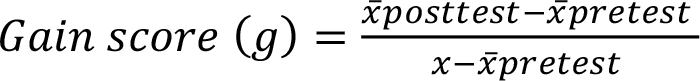(1)

If gain score (g) ≥ 0,7 then it is in the high category, if 0,7 > (g) ≥ 0,3 then it is in medium category, and if gain score (g) < 0,3 then it is in the low category. Furthermore, revealing the effectiveness of the use of MIKIMOM used the statistical analysis applying the MANOVA test applying IBM SPSS. The first decision was made based on the output of SPSS on Hotelling Trace. The higher the score on Hotelling Trace the bigger the effect of the media. Revealing the effect size of MIKIMOM on the improvement of the students’ critical thinking and mathematical representation abilities was conducted through the analysis of the effect size using multivariate GLM (General Linear Model). The GLM output in the form of eta square was converted into the value of Cohen’s f so as to result in the effect size. The value of the effect size can be calculated with the equation (Cohen, 1988).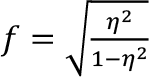(2)
 Cohen’s f Interpretation 0.0 ≤ f < 0.10 Small Effect Size 0.10 ≤ f < 0.25 Medium Effect Size 0.25 ≤ f < 0.4 Large Effect Size 0.4 ≤ f Very Large Effect Size

Table 4. Interpretation of Cohen’s value

However, prior to the analysis of MANOVA statistics, there needed to be the statistic prerequisite analysis. The prerequisite analysis consisted of the multivariate normality analysis using Shapiro-Wilk, correlation analysis using Pearson Correlation, and homogeneity analysis using Box’s M test.

3. Findings and Discussion

3.1. Reliability and Validity of Critical Thinking and Mathematical Representation Ability Test

The reliability of the critical thinking and mathematical representation ability test based on the QUEST output is good. The reliability coefficient is shown in the value of the summary of item estimates and summary of case estimates which ranges from 0.6 to 0.8 as shown in Table 5.

All of the test items are not only reliable but also valid because they are fit to PCM. This can be seen in the value of the infit mean square which ranges from 0.70 to 1.30 and falls into the valid category, as shown in Figures 3 and 4.

 No Items tested Summary of item estimate Summary of case estimate 1 Critical thinking ability 0.81 0.69 2 Mathematical representation ability 0.79 0.67

Table 5. Result of the analysis of the reliability of critical thinking and mathematical representation ability test items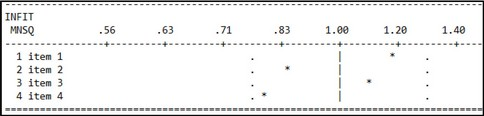Figure 3. Result of analysis of item fitness of critical thinking ability test items

Based on the “it.out” of QUEST which aims to reveal the fitness of each item to IRT, all of the test items can be used for the assessment instrument, as shown in Table 6.

Therefore, the items of the essay test in both critical thinking ability and mathematical representation ability are valid and reliable, and they can be used for assessing the improvement in the students’ critical thinking and mathematical representation abilities.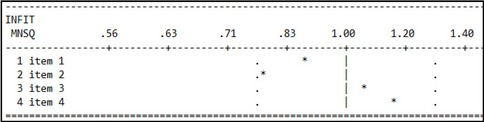Figure 4. Result of analysis of item fitness of mathematical representation ability

 Items Item name INFIT MNSQ Criteria OUFIT t Criteria DIFFCLTY Criteria Item quality Critical thinking Item 1 1.17 Fit 1.6 Valid -0.80 Medium Good Item 2 0.85 Fit -1.8 Valid -0.23 Medium Good Item 3 1.10 Fit 0.6 Valid 0.35 Medium Good Item 4 0.79 Fit -2.6 Valid 0.68 Medium Good Mathematical representation Item 1 0.88 Fit -1.4 Valid -0.18 Medium Good Item 2 0.78 Fit -2.3 Valid 0.69 Medium Good Item 3 1.06 Fit 0.2 Valid 0.28 Medium Good Item 4 0.16 Fit 1.8 Valid -0.79 Medium Good

Table 6. Interpretation of test items

3.2. Improvement in Critical Thinking and Mathematical Representation Abilities

Improvement in critical thinking and mathematical representation abilities can be seen in the students’ test result before and after they were given treatment using MIKIMOM and PPT. The test result was then analyzed for statistic prerequisite to see the normality, homogeneity, and correlation before the statistical analysis.

Based on the result of the normality test, all of the data from the population are normally distributed. All of the data are on the value of .sig > 0.05 in the column of Shapiro-Wilk in Table 7.

 Class Ability Shapiro-Wilk Statistic Df Sig. Experimental Critical thinking Pretest 0.153 32 0.061 Mathematics Pretest 0.144 32 0.074 Critical thinking Posttest 0.149 32 0.078 Mathematics Posttest 0.149 32 0.075 Control Critical thinking Pretest 0.152 32 0.209 Mathematics Pretest 0.163 32 0.060 Critical thinking Posttest 0.156 32 0.189 Mathematics Posttest 0.150 32 0.195

Table 7. Result of normality test

The data are not only normally distributed, but also homogeneous. This is shown by the value of .sig 0.254 in Table 8. This value is > 0.05 and thus it can be said that there is no variant difference between the control class and the experimental class so that the data are homogeneous.

The value of sig. (2-tailed) in Table 9 is 0.000, which is < 0.05. Therefore, it can be said that there is a relationship between mathematical representation and critical thinking abilities. Pearson correlation coefficient is 0.554, which lies between 0.40 and 0.599 and thus the relationship between the two variables is quite strong.

The prerequisite analyses were followed by the analyses of the improvement and effectiveness of the use of comics. The result of the statistical test of MANOVA on Hotteling’s Trace shows that the value of sig is 0.000, which is lower than 0.05.

Therefore, it can be said that there is a significant difference in the average score of the improvement of critical thinking and mathematical representation abilities between the class taught by using the MIKIMOM and that taught by using PPT. The improvement in the critical thinking and mathematical representation abilities can be seen in Table 11 and 12.

 Box’s test of equality of covariance matrices Box’s M 4.220 F 1.357 df1 3 df2 691920.000 Sig. 0.254

Table 8. Result of homogeneity test

 Correlation Mathematical representation Critical thinking Mathematical representation Pearson Correlations 1 0.554 Sig. (2-tailed) 0.000 N 64 64 Critical thinking Pearson Correlations 0.554 1 Sig. (2-tailed) 0.000 N 64 64

Table 9. Result of correlation test

 Effect Value Sig. Hotelling’s Trace 0.321 0.000

Table 10. Result of MANOVA

 No Class Number of students Average of pretest Average of postest Average of gain score Category 1 Eksperimental 32 28.13 80.86 0.73 High 2 Control 32 30.47 69.53 0.56 Medium

Table 11. Result of improvement in critical thinking abilities

 No Class Number of students Average of pretest Average of postest Average of gain score Category 1 Eksperimental 32 32.42 86.13 0.79 High 2 Control 32 25.98 70.31 0.61 Medium

Table 12. Result of improvement in mathematical representation abilities

 No Variable Eta square Cohen’s f Interpretation 1 Critical thinking 0.179 0.197 Medium Effect Size 2 Mathematical representation 0.287 0.339 Large Effect Size

Table 13. Result of analysis of effect size

Table 11 and 12 shows that both the critical thinking ability and mathematical representation ability are in the high category in the experimental class. The comic media is effective in improving critical thinking and mathematical representation abilities. The effect size can be seen in the result of the analysis of effect size in Table 13. Column Eta Square shows the scores of 0.287 (large effect size) and 0.179 (medium effect size). Therefore, it can be said that MIKIMOM is effective in improving critical thinking and mathematical representation abilities with the medium and large effect size.

Table 13 has similarity with the research by (Sari, Nikmah, Kuswanto & Wardani, 2020) which reported that the teaching by using a comic integrated to traditional games or local wisdom was effective and could improve students’ mathematical representation ability. The research by (Azmy, Kuswanto & Susetyo, 2020) also reported that teaching by using comic media could improve students’ critical thinking ability.

Table 13 shows that there is a difference in the improvement both the critical thinking ability and mathematical representation ability of the experimental class and control class. This shows that the experimental class which used the MIKIMOM is better at the improvement in critical thinking and mathematical representation abilities compared to the conventional class. One of the factors affecting this is the characteristic of the media. The comic media contains the teaching of mathematical representation which facilitates students to understand a certain concept (Supandi et al., 2018) and solve problems by using their reasoning skill (Nor’ain Mohd & Chinnappan, 2016). It contains indicators of critical thinking which can encourage students to reason, discuss, and draw conclusions by themselves (Viennot & Nicolas, 2018) which results in their high academic achievement (Alessio, Avolio & Charles, 2019). In addition, the media refers to learning everyday life phenomena such as carom games, so that critical thinking ability can be improved (Yuliati et al., 2018). Comic media is also integrated to discovery learning, the teaching model which can stimulate and facilitate students to think critically (Anggraini, Murni & Sakur, 2018; Sulistiani et al., 2018) and help improve mathematical representation ability (Minarni et al., 2016; Ertikanto, Rosidin, Distrik, Yuberti & Rahay 2018).

The MIKIMOM enables students to learn individually because it contains the steps of discovery learning. Students can learn at anytime and anywhere. They can understand the learning materials easily because the materials are related to everyday life phenomena in carom games. The simple colloquial language makes students not easily get bored of reading the comic materials and content. In addition, students can stimulate critical thinking and mathematical representation abilities through the conversation among the characters in the comic, which contains indicators of critical thinking and mathematical representation abilities. These two abilities can also be improved by doing discussion items in the comic and then evaluating the abilities from the available discussion.